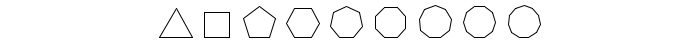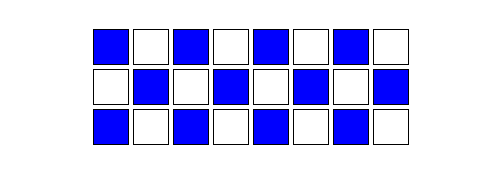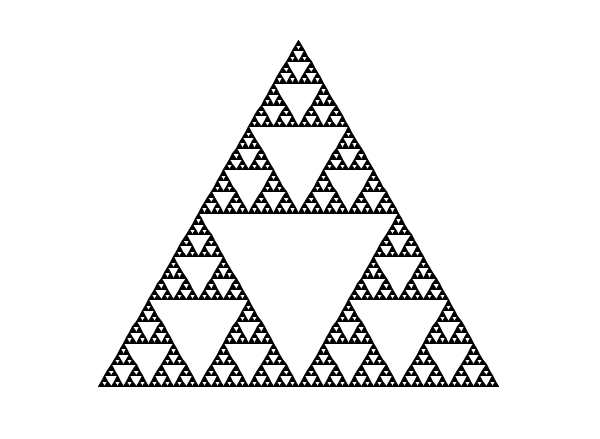# Basic C programming

The goal of this tutorial is to learn basic C programming constructs. We will do this by examining some of the familiar constructs from Java and learning their C equivalents.

In order to make things more interesting than simply printing out "Hello world." like we did in the last class, we will be using a basic bitmap drawing package base on turtle graphics.Row of Shapes

## Learning Objectives

By the end of this lab, you should be able to:

• Write basic C programs using standard constructs.
• Perform basic formatted output.
• Write basic C functions.
• Generate images using turtle graphics.

## Starter Code

IN THE LINUX LAB (ISAT 250): Download lab02.tar.gz and untar it from the command line with the following command from the folder that you saved the file in:

``tar -zxvf lab02.tar.gz``

IN THE MAC LAB (ISAT 248): Download lab02.zip and unzip it by either double-clicking it or using the following command line command in the Terminal from the folder that you saved the file in:

``unzip lab02.zip``

The starter code has four files: a `main.c`, which you will be modifying; `turtle.h` and `turtle.c`, which contain the turtle graphics library; and an OS-specific `Makefile` (the latter is why it is important to download the correct file for your lab).

Open up the `main.c` file, and add the following lines to `int main()` where it says `// TODO: add your code here`:

``````turtle_forward(100);
turtle_turn_left(90);
turtle_forward(100);
turtle_draw_turtle();``````

Now compile and run the `main` program. You'll see that it produced an output file, `output.bmp`. Open this in an image viewer:

IN THE LINUX LAB (ISAT 250): Use the following command from the project folder:

``eog output.bmp``

IN THE MAC LAB (ISAT 248): Double-click the bitmap file in Finder or use the following command in the Terminal from the project folder:

``open output.bmp``

The `turtle.h` contains complete documentation for the functions available to you in the turtle library. The following functions should be enough for today's lab:

• `turtle_forward(int distance)` / `turtle_backward(int distance)`

Move the turtle forwards/backwards by the number of pixels indicated.

• `turtle_turn_left(double angle)` / `turtle_turn_right(double angle)`

Rotate the turtle left/right by `angle` degrees.

• `turtle_pen_up()` / `turtle_pen_down()`

After `turtle_penup()` is called the turtle will not draw until `turtle_pendown()` is called.

• `turtle_goto(int x, int y)`

Go to the `(x, y)` position indicated. Note that `(0,0)` is the center of the screen and this command preserves the orientation (direction) of the turtle.

• `turtle_set_heading(double angle)`

Set the turtle's orientation to the indicated angle. Zero degrees points towards the right, and ninety degrees points up.

• `turtle_init(int w, int h)`

Initializes the image size of the turtle's space to width x height = `(w, h)`.

• `turtle_save_bmp(char* filename)`

Saves the computed image to a file specified by the filename.

To learn more about the turtle library, see the reference page.

### Getting Started

• Spend a few minutes experimenting with compiling the code. Make changes to `main.c` to draw different things using the commands listed above. Can you make the turtle draw a square? What about a rectangle or a triangle?

## Java to C: A Crash Course

### Data types

C has multiple integer data types; the most common is `int`, which is sufficient for our current needs. It is a signed integer at least 16 bits wide. On most modern architectures, it is at least 32 bits.

You can read about the other integer data types at the wikipedia page. In future labs, we will use the `size_t` type, which is used by convention when we want to store non-negative size values.

Like Java, C has two floating-point formats: the 32-bit `float` and the 64-bit `double`.

Like Java, C also has a single character data type called `char`. However, unlike Java, C does not have a built-in string type. Rather, strings are conventionally stored as an array of `char` values. We will discuss strings in C next week.

Finally, if you `#include <stdbool.h>`, you may use the `bool` data type, which is the same as the `boolean` data type from Java. The `bool` data type is not part of the original C specification; it was a convention to use integers instead (0 for false and 1 for true).

Like Java, C has two kinds of comments: a single line format and a multi-line format. Here are examples of both:

``````c = a + b;          // this is a single line comment

/* this is a
* multi-line comment */
d = a * b;``````

### Console output: Using `printf`

The C standard library contains a very powerful string output function called `printf`; it can handle many different kinds of output formats. For the purposes of this class, we will only need a few features.

The `printf` function is rather unusual in that it can take a variable number of parameters. The first parameter is always a string, often called the "format string." If all you want to do is print a simple string, that's all you have to pass:

``printf("Hello!\nThis is some simple text.");``

The resulting output is:

``````Hello!
This is some simple text.``````

There are several important escape sequences:

Code Description
`\n` newline
`\r` tab
`\"` quote mark

To print the values of variables, you can embed format specifiers into the format string. For example:

``````int a = 42;
float pi = 3.141592;
printf("The answer is %d and %s is approximately %f.\n", a, "PI", pi);``````

The resulting output is:

``The answer is 42 and PI is approximately 3.141592.``

Here is a list of important format specifiers:

Code Description
`%d` signed integer (`int`)
`%lu` unsigned long integer (`size_t`)
`%f` floating-point number (`float` or `double`)
`%e` scientific notation (`float` or `double`)
`%c` character (`char`)
`%s` character string (`char[]`)
`%p` pointer (we will see these later!)

There is no format specifier for the `bool` data type. If you wish to output the value of a boolean, you will need to use a conditional to print different strings (perhaps `"true"` and `"false"`).

Here is a full reference to all the capabilities of the `printf` function.

### Conditionals

Conditional statements in C look exactly like their Java counterparts.

``````if (some-test) {
...
} else if (a-second-test) {
...
} else {
...
}``````

### Functions, parameters, calling, and recursion

Function definitions in C look very similar to their Java counterparts. For example:

``````int add(int a, int b) {
return a + b;
}``````

In this example, the function `add` takes two integer parameters, `a` and `b` and returns an their sum as an integer.

One difference between Java and C is that in Java every "function" is a method of some class. So the `add` function defined above would be part of some class and to call it, you would need an object of that class. Something like:

``int result = obj.add(3, 4); // add 3 and 4 using the add method of obj``

C, however, is not object oriented. Functions are not part of some parent object, so you call them directly:

``int result = add(3, 4); // add 3 and 4 using the add function``

### Exercise: Drawing Rectangles

• Add a new function with the following signature:

`void rectangle(int x, int y, int width, int height);`

This function should draw a rectangle with the indicated width and height. The lower-left corner of the rectangle should be at position `(x, y)`.

• Call your function from inside `main()`. Test it by recompiling and running the application from the command line.

Question: Can you define the function after `main()`?

### Looping: `for` and `while`

As with Java, the main looping constructs in C are the `for` and `while` loops. Unlike Java, there is only one syntax for the `for` loop, namely:

``````for (int i = 0; i < n; i++) {
...
}``````

or more generally,

``````for (initialization; condition; update) {
...
}``````

The `while` loop behaves exactly the same as in Java:

``````while (something_is_true) {
do_this();
}``````

### Exercise: Rows

• Add a new function with the following signature:

`void row(int x, int y, int count, int size);`

This function should draw a horizontal row of squares with the lower-left corner of the row at position `(x, y)`. The `count` and `size` parameters indicate the number of squares in the row and the size of each square respectively. Your function should leave a small (5-10 pixel) fixed space between each square.

Do not copy/paste code from the existing `rectangle` function! Your `row` function should invoke `rectangle` with appropriate parameter values.

• Update the `main` function so that it includes several calls to your newly defined `row` function.

## Optional Exercises

### Polygons

• Add a new function with the following signature:

`void polygon(int x, int y, int num_sides, int size);`

This function should draw a regular polygon with the indicated number of sides starting at position `(x, y)`. The length of each side is determined by the `size` parameter. This function should work for any value of `sides` that is greater than 2. Do not hard-code the function for any particular number of sides.

Hint: * This can be accomplished using either a `for` or a `while` loop. * Note that the turning angles required to draw a square are all `90` degrees and that `90 = 360 / 4`.

• Update the `main` function so that it draws several different polygons in different places with different numbers of sides and side lengths.
• Challenge Goal: Try writing code to draw a series of polygons with the same general size but with an increasing number of sides (as in the graphic at the top of this page). You need to change the side length of the polygons as you increase the number of sides. Here are some questions to think about:
• Do you need to increase or decrease the side length as you increase the number of sides.
• After how many sides is the polygon indistinguishable from a circle?
• Does this number vary if you change the initial size?

### Grids

• Write a new function with the following signature:

`void grid(int x, int y, int columns, int size)`

This function should create a `rows x columns` grid of squares each of size `size` with the lower-left corner at `(x, y)`. The `size` parameter indicates teh width of the individual squares.

• For further experimentation, make alternating squares filled in a checkerboard pattern. You will need to look through the documentation for the `turtle_begin_fill()` and `turtle_end_fill()` functions. Here is an example of what it could look like:A grid of alternating color blocks

• Update the `main` function so that it includes several calls to your newly defined `grid` function.

### Random Walks

Here is some code that simulates rolling a single die, generating a single random number in the range [0,5]:

``````#include <time.h>
#include <stdlib.h>

/*
* returns a random integer from 0 to 5 (inclusive)
*/
int roll_dice()
{
static int initialized = 0;
if (!initialized) {
srand(time(NULL));
initialized = true;
}
return rand() % 6;
}``````

Don't worry about the details of how that function works right now; just copy-and-paste it into your `main.c` above the `main()` function declaration. Now write a function called `turtle_random_walk` that does a random walk. It should take an integer parameter telling the turtle how many steps to take.

For each step, the function should call the `roll_dice` function to generate a random number. If the number is 0 or 1, the turtle should move forward 10 spaces. If the number is 2 or 3, the turtle should turn left 90 degrees. If the number is 4 or 5, the turtle should turn right 90 degrees.

Run your program several times and observe the results.

### Challenge Problem: Sierpinski

If you want some practice with a more complex algorithm, try writing a function that draws a Sierpinski triangle. You should be able to reuse the polygon function you wrote earlier. Here is an example of what the end result should look like:A Sierpinski triangle

Hint: Use recursion =D.